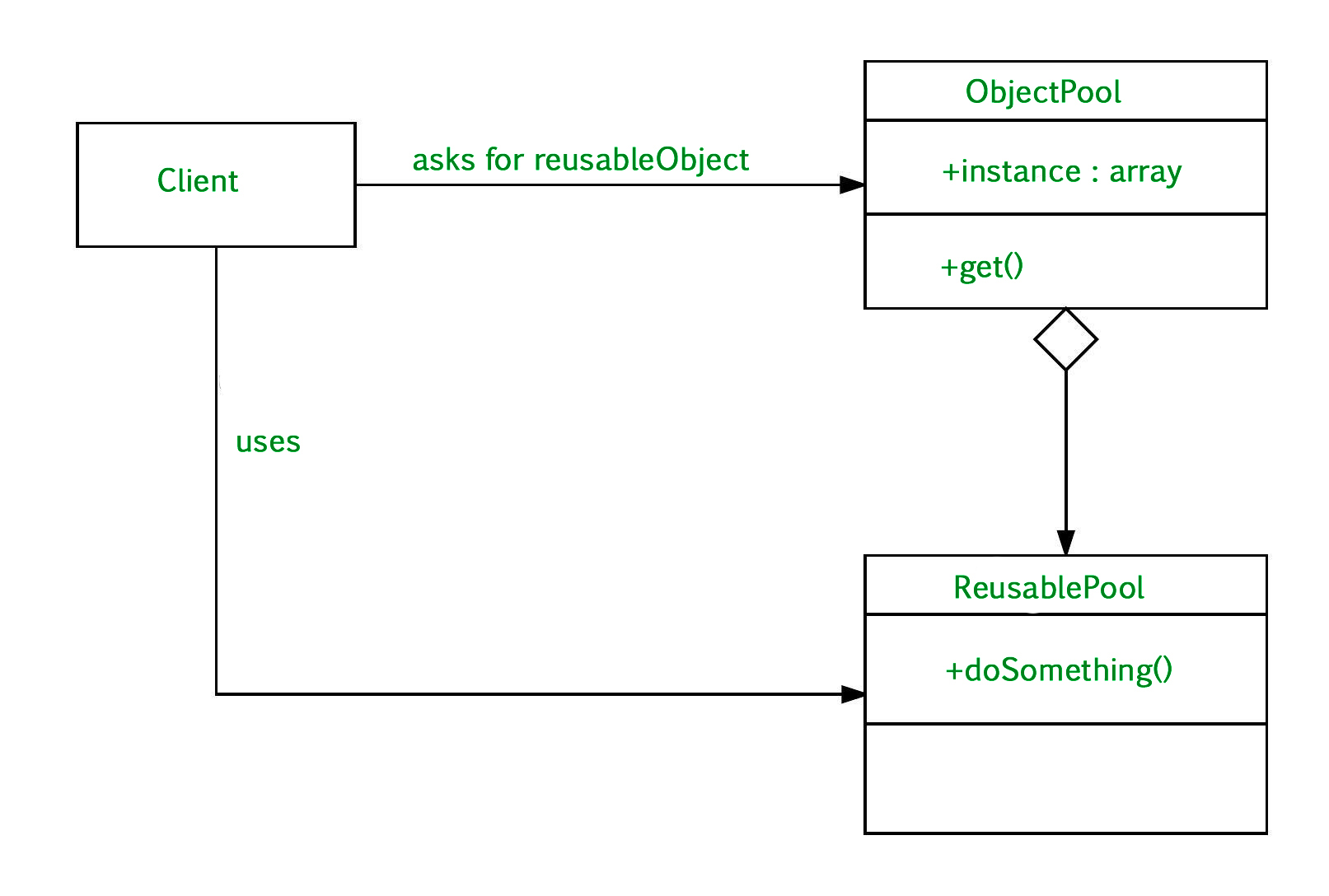# Object Pool Design Pattern

Object pool pattern is a software creational design pattern which is used in situations where the cost of initializing a class instance is very high.
Basically, an Object pool is a container which contains some amount of objects. So, when an object is taken from the pool, it is not available in the pool until it is put back.
Objects in the pool have a lifecycle:

• Creation
• Validation
• Destroy.

UML Diagram Object Pool Design Pattern• Client : This is the class that uses an object of the PooledObject type.
• ReuseablePool: The PooledObject class is the type that is expensive or slow to instantiate, or that has limited availability, so is to be held in the object pool.
• ObjectPool : The Pool class is the most important class in the object pool design pattern. ObjectPool maintains a list of available objects and a collection of objects that have already been requested from the pool.

Let’s take the example of the database connections. It’s obviously that opening too many connections might affect the performance for several reasons:

• Creating a connection is an expensive operation.
• When there are too many connections opened it takes longer to create a new one and the database server will become overloaded.

Here the object pool manages the connections and provide a way to reuse and share them. It can also limit the maximum number of objects that can be created.

 `// Jave program to illustrate ` `// Object Pool Design Pattern ` `abstract` `class` `ObjectPool { ` `    ``long` `deadTime; ` ` `  `    ``Hashtable lock, unlock; ` ` `  `    ``ObjectPool() ` `    ``{ ` `        ``deadTime = ``50000``; ``// 50 seconds ` `        ``lock = ``new` `Hashtable(); ` `        ``unlock = ``new` `Hashtable(); ` `    ``} ` ` `  `    ``abstract` `T create(); ` ` `  `    ``abstract` `boolean` `validate(T o); ` ` `  `    ``abstract` `void` `dead(T o); ` ` `  `    ``synchronized` `T takeOut() ` `    ``{ ` `        ``long` `now = System.currentTimeMillis(); ` `        ``T t; ` `        ``if` `(unlock.size() > ``0``) { ` `            ``Enumeration e = unlock.keys(); ` `            ``while` `(e.hasMoreElements()) { ` `                ``t = e.nextElement(); ` `                ``if` `((now - unlock.get(t)) > deadTime) { ` `                    ``// object has deadd ` `                    ``unlock.remove(t); ` `                    ``dead(t); ` `                    ``t = ``null``; ` `                ``} ` `                ``else` `{ ` `                    ``if` `(validate(t)) { ` `                        ``unlock.remove(t); ` `                        ``lock.put(t, now); ` `                        ``return` `(t); ` `                    ``} ` `                    ``else` `{ ` `                        ``// object failed validation ` `                        ``unlock.remove(t); ` `                        ``dead(t); ` `                        ``t = ``null``; ` `                    ``} ` `                ``} ` `            ``} ` `        ``} ` `        ``// no objects available, create a new one ` `        ``t = create(); ` `        ``lock.put(t, now); ` `        ``return` `(t); ` `    ``} ` `    ``synchronized` `void` `takeIn(T t) ` `    ``{ ` `        ``lock.remove(t); ` `        ``unlock.put(t, System.currentTimeMillis()); ` `    ``} ` `} ` ` `  `// Three methods are abstract ` `// and therefore must be implemented by the subclass ` ` `  `class` `JDBCConnectionPool ``extends` `ObjectPool { ` `    ``String dsn, usr, pwd; ` ` `  `    ``JDBCConnectionPool(String driver, String dsn, String usr, String pwd) ` `    ``{ ` `        ``super``(); ` `        ``try` `{ ` `            ``Class.forName(driver).newInstance(); ` `        ``} ` `        ``catch` `(Exception e) { ` `            ``e.printStackTrace(); ` `        ``} ` `        ``this``.dsn = dsn; ` `        ``this``.usr = usr; ` `        ``this``.pwd = pwd; ` `    ``} ` ` `  `    ``Connection create() ` `    ``{ ` `        ``try` `{ ` `            ``return` `(DriverManager.getConnection(dsn, usr, pwd)); ` `        ``} ` `        ``catch` `(SQLException e) { ` `            ``e.printStackTrace(); ` `            ``return` `(``null``); ` `        ``} ` `    ``} ` ` `  `    ``void` `dead(Connection o) ` `    ``{ ` `        ``try` `{ ` `            ``((Connection)o).close(); ` `        ``} ` `        ``catch` `(SQLException e) { ` `            ``e.printStackTrace(); ` `        ``} ` `    ``} ` ` `  `    ``boolean` `validate(Connection o) ` `    ``{ ` `        ``try` `{ ` `            ``return` `(!((Connection)o).isClosed()); ` `        ``} ` `        ``catch` `(SQLException e) { ` `            ``e.printStackTrace(); ` `            ``return` `(``false``); ` `        ``} ` `    ``} ` `} ` ` `  `class` `Main { ` `    ``public` `static` `void` `main(String args[]) ` `    ``{ ` `        ``// Create the ConnectionPool: ` `        ``JDBCConnectionPool pool = ``new` `JDBCConnectionPool( ` `            ``"org.hsqldb.jdbcDriver"``, ``"jdbc:hsqldb: //localhost/mydb"``, ` `            ``"sa"``, ``"password"``); ` ` `  `        ``// Get a connection: ` `        ``Connection con = pool.takeOut(); ` `        ``// Return the connection: ` `        ``pool.takeIn(con); ` `    ``} ` `} `

• It offer a significant performance boost.
• It manages the connections and provides a way to reuse and share them.
• Object pool pattern is used when the rate of initializing a instance of the class is high.

When to use Object Pool Design Pattern

• When we have a work to allocates or deallocates many objects
• Also, when we know that we have a limited number of objects that will be in memory at the same time.

Reference :

https://sourcemaking.com/design_patterns/object_poolMy Personal Notes arrow_drop_upCheck out this Author's contributed articles.

If you like GeeksforGeeks and would like to contribute, you can also write an article using contribute.geeksforgeeks.org or mail your article to contribute@geeksforgeeks.org. See your article appearing on the GeeksforGeeks main page and help other Geeks.

Please Improve this article if you find anything incorrect by clicking on the "Improve Article" button below.

Article Tags :
Practice Tags :

2

Please write to us at contribute@geeksforgeeks.org to report any issue with the above content.